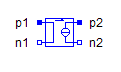From Space Phasor - MapleSim Help

From Space Phasor

Convert a space phasor to a multiphase complex signalDescription The From Space Phasor component transforms a space phasor to a quasistationary multiphase signal.Equations ${y}_{k}=\frac{\sqrt{2}}{2}\left({u}_{1}+j{u}_{2}\right)\mathrm{exp}\left(-j{\phi }_{k}\right)$Connections

 Name Description Modelica ID $u$ Space phasor input u $y$ Complex vector output yParameters

 Name Default Units Description Modelica ID $m$ $3$ Number of phases mModelica Standard Library The component described in this topic is from the Modelica Standard Library. To view the original documentation, which includes author and copyright information, click here.